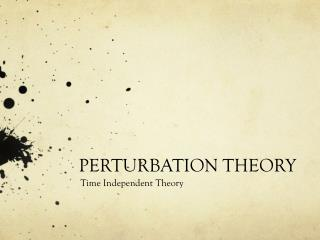DownloadDownload PresentationPERTURBATION THEORY

# PERTURBATION THEORY

Download Presentation## PERTURBATION THEORY

- - - - - - - - - - - - - - - - - - - - - - - - - - - E N D - - - - - - - - - - - - - - - - - - - - - - - - - - -
##### Presentation Transcript

1. PERTURBATION THEORY Time Independent Theory

2. A Necessity: • Exactly solvable problems are very few and do not really represent a real physical system completely. • In many cases, the primary interaction on the system maybe exactly solvable and is hence the dominant contribution. • We develop a theory which takes the exact solution as a base to build on, for more accurate description of the system.

3. Recipe of the Theory • Take the Hamiltonian of an exactly solvable problem. • Add a perturbative term to the original Hamiltonian, satisfying the condition, The terms in the perturbation need to be smaller than the energy differences of the original Hamiltonian. • Introduce a parameter l as the co-efficient of the perturbative term in the Hamiltonian, to observe the effect of the Hamiltonian.

4. Eigen Value changes with perturbation intensity • Observation: Start with a negligible value of l such that the perturbation is very minuscule to start with. This causes the change in the Eigen-values and functions to vary slightly from the original Hamiltonian. Smoothly varying the parameter l smoothly varies the new solution as well.

5. Taylor Expansion • The implication is, the existence of a continuous Eigen-functions and Eigen-values: and for an Hamiltonian parameterized by l as • Hence for small values of perturbation, the solutions can be expanded in Taylor series around the known solution with l = 0, as for the eigen-value equation: The original equation: